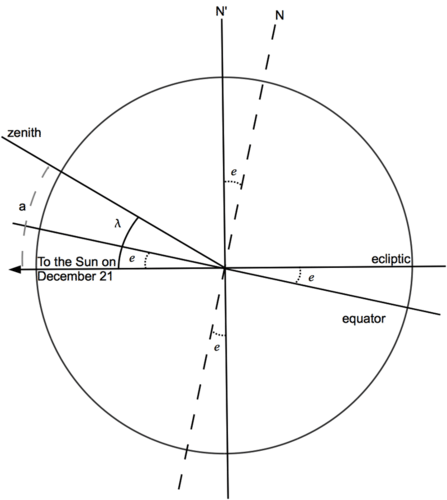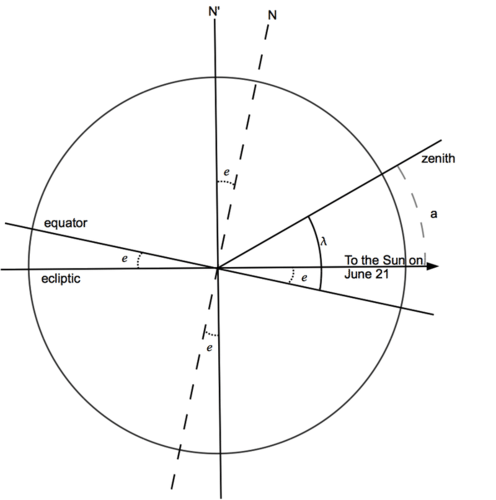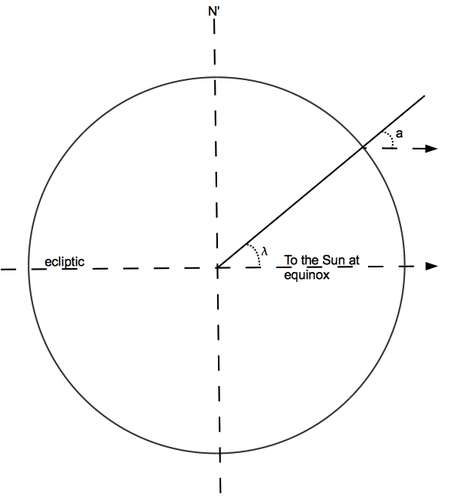# 11.3: Finding Latitude with the Noontime Sun

•• Contributed by CK12
• CK12

If you are sailing a ship in mid-ocean, you can get the same information from the noontime Sun --- probably more accurately, since at night you might not see the horizon very well.

Noon is when the Sun reaches the highest point in its journey across the sky. It then crosses the north-south direction--in the northern hemisphere, usually south of the observer. Because the axis of the Earth is inclined by an angle $$e$$=23.5 to a line perpendicular to the ecliptic, the height of that point above the horizon depends on the season. Suppose you are at point $$P$$. We examine 3 possibilities:

(1) Suppose the date is the winter solstice, around December 21, when the north pole is inclined away from the Sun. To find your latitude $$\lambda$$ you measure the angle a between the direction of the noontime Sun and the zenith.Figure $$\PageIndex{1}$$: Position of the noon Sun at the winter solstice.

Look at the drawing and imagine you could rotate the equator and the north pole $$N$$ until they reached the ecliptic and the pole of the ecliptic $$N′$$. Then all three angles marked e fold up together, showing that they are equal. You get

$a\,=\lambda \,+ e$

$\lambda\,=\,a\,-\,e\,=\,a\,-\,23.5^∘$

(2) Half a year later, at the summer solstice (June 21), the north pole is inclined towards the Sun, not away from it, and now (if $$\lambda$$ is larger than $$e$$)Figure $$\PageIndex{2}$$: Position of the noon Sun at the summer solstice.

$a\,=\lambda\,-\,e$

$\lambda\,=\,a\,+\,e\,=\,a\,=\,23.5^∘$

(3) Finally, suppose you are at equinox, around March 21 or September 21. The inclination of the Earth's axis is now out of the plane of the drawing --- away from the paper, if this were a picture in a book.Figure $$\PageIndex{3}$$: Position of the noon Sun at equinox.

The direction to the Sun is in the plane of the equator, and we get

$\lambda\,=\,a$

Thus at least at those dates, seafarers could tell what their latitude was by measuring the position of the noontime Sun.

For any other date, navigation tables exist that give the proper angle (smaller than 23.5 degrees) which must be added or subtracted. They also provide formulas for deriving the height of the noontime Sun from observations made at other times.

As with the pole star, rather than measuring the angle a from the zenith --- which is not marked in the sky! --- it is simpler to measure the angle (90°–$$a$$) from the horizon, which at sea is usually sharply defined. Such observations, known as “shooting the Sun," are done with an instrument known as the sextant. It has a sliding scale covering 1/6 of a circle (hence the name) and an attached pivoted mirror, providing a split view: by moving the scale, the sea-officer brings Sun and horizon simultaneously into view and then reads off the angle between them.